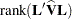Testing Effects

For each effect in the model, PROC SURVEYREG  computes anmatrix such that every element ofis estimable; thematrix has the maximum possible rank that is associated with the effect. To test the effect, the procedure uses the Wald F statistic for the hypothesis. The Wald F statistic equalswith numerator degrees of freedom equal to.

In the Taylor series method, the denominator degrees of freedom is equal to the number of clusters minus the number of strata (unless you specify the denominator degrees of freedom with the DF= option in the MODEL statement). For details about denominator degrees of freedom in replication methods, see the section Denominator Degrees of Freedom. It is possible that thematrix cannot be constructed for an effect, in which case that effect is not testable. For more information about how the matrixis constructed, see the discussion in Chapter 15, The Four Types of Estimable Functions.

You can use the TEST statement to perform F tests that test Type I, Type II, or Type III hypotheses. For details about the syntax of the TEST statement, see the section TEST Statement of Chapter 19, Shared Concepts and Topics.